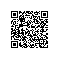# 《Java从小白到大牛精简版》之第6章 运算符（下）

## 6.4 位运算符

[图片上传失败...(image-a9ef68-1511744132099)]

byte a = 0B00110010;    //十进制50                 ①
byte b = 0B01011110;    //十进制94                 ②

System.out.println("a | b = " + (a | b));   // 0B01111110   ③
System.out.println("a & b = " + (a & b));   // 0B00010010   ④
System.out.println("a ^ b = " + (a ^ b));   // 0B01101100   ⑤
System.out.println("~b = " + (~b));         // 0B10100001   ⑥

System.out.println("a >> 2 = " + (a >> 2));     // 0B00001100   ⑦
System.out.println("a >> 1 = " + (a >> 1));     // 0B00011001   ⑧
System.out.println("a >>> 2 = " + (a >>> 2));   // 0B00001100   ⑨
System.out.println("a << 2 = " + (a << 2));     // 0B11001000   ⑩
System.out.println("a << 1 = " + (a << 1));     // 0B01100100   ⑪

int c = -12;                                    ⑫
System.out.println("c >>> 2 = " + (c >>> 2));   ⑬
System.out.println("c >> 2 = " + (c >> 2));     ⑭

a | b = 126
a & b = 18
a ^ b = 108
~b = -95
a >> 2 = 12
a >> 1 = 25
a >>> 2 = 12
a << 2 = 200
a << 1 = 100
c >>> 2 = 1073741821
c >> 2 = -3

*提示 有符号右移n位，相当于操作数除以2<sup>n</sup>，例如代码第⑦行(a >> 2)表达式相当于(a / 2<sup>2</sup>)，a = 50所以结果等于12，类似的还有代码第⑧行和第⑭行。另外，左位移n位，相当于操作数乘以2<sup>n</sup>，例如代码第⑩行(a << 2)表达式相当于(a 2<sup>2</sup>)，a = 50所以结果等于200，类似的还有代码第⑪行。**

## 6.5 其他运算符

• 三元运算符（? :）。例如x?y:z;，其中x、y和z都为表达式。
• 小括号。起到改变表达式运算顺序的作用，它的优先级最高。
• 中括号。数组下标。
• 引用号（.）。对象调用实例变量或实例方法的操作符，也是类调用静态变量或静态方法的操作符。
• 赋值号（=）。赋值是用等号运算符（=）进行的。
• instanceof。判断某个对象是否为属于某个类。
• new。对象内存分配运算符。
• 箭头（->）。Java 8新增加的，用来声明Lambda表达式。
• 双冒号（::）。Java 8新增加的，用于Lambda表达式中方法的引用。

import java.util.Date;

public class HelloWorld {

public static void main(String[] args) {

int score = 80;
String result = score > 60 ? "及格" : "不及格"; // 三元运算符（? : ）
System.out.println(result);

Date date = new Date();         // new运算符可以创建Date对象
System.out.println(date.toString());    //通过.运算符调用方法

}
}

## 6.6 运算符优先级

[图片上传失败...(image-34534b-1511744132099)]

## 本章小结使用钉钉扫一扫加入圈子
+ 订阅